Last updated 13 Feb 2018

CHEMISTRY IN PERSPECTIVE
(an electrostatic approach for bored and confused A-level chemistry
or other senior school chemistry students)

SEND EMAIL
HOME

Recommended by:Top 20 UK science resources,
The Tutor Website

Recommended by:Rated:2010

Listed on the science, engineering and technology section of'providing you with access to the very best Web resources for education and research, evaluated and selected by a network of subject specialists.'
(Please note that intute closed in July 2011)

Section 2: Inorganic

CHAPTER 15: GROUPS I and II
NB This chapter has now been updated to improve browser compatibility.

15.1. INTRODUCTION

15.1.1. The order of these next three chapters may seem unusual: groups I and II, then group VII, then group IV. It is quite intentional. The chemistry of groups I and II is predominated by the low effective nuclear charge of the elements they contain. The chemistry of group VII is predominated by the high effective nuclear charge of the elements it contains. It is useful to look at these two extremes before considering group IV as an example of a group where the effective nuclear charge is intermediate, and the chemistry changes down the group from being more like group VII to being more like groups I and II.

15.1.2. The elements in groups I and II are all very similar. Their low effective nuclear charge means that their chemistry is dominated by the loss of electrons to form positive ions (section 4.4.2.). Group I elements form 1+ ions and group II elements form 2+ ions (section 4.7.4.).

There are some differences between groups I and II, mainly due to the higher effective nuclear charge in group II. There are also changes in behaviour down both groups due to the increase in number of shells. Since the dominating effect of low effective nuclear charge is less marked in group II, the changes are more noticeable down group II.

The changes down groups I and II, and the differences between them, form the basis of this chapter, but do not get them out of proportion. The overwhelming characteristic of these elements is their similarity to each other. They are all electropositive metals which nearly all form basic oxides and hydroxides.

15.2. THE ELEMENTS

15.2.1. Atomic and ionic radius increase down both groups as can be predicted from the increasing number of shells (see 4.2.2). The higher effective nuclear charge in group II enables us to predict that the radii of both atoms and ions will be lower in group II.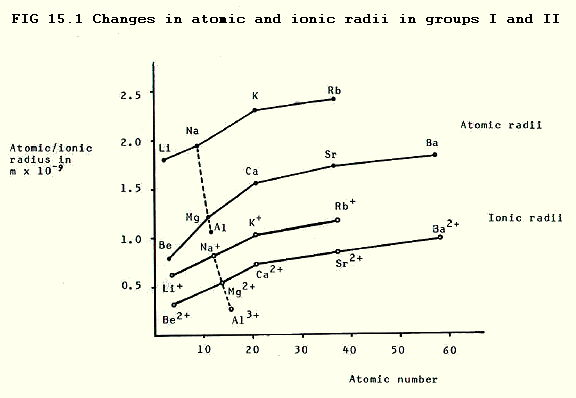In addition, the ions are smaller than the parent atoms, not only because they have one less shell containing electrons, but also because the ratio of protons to electrons is higher and thus the effective nuclear charge is higher in the ions. Note that this increase in effective nuclear charge is greater when group II elements form ions.

15.2.2. Metallic bond strength decreases down both groups because the nuclei become further from the metallic bonding orbital of electrons. In group II the higher effective nuclear charge, and the greater number of electrons in the metallic bonding orbital, enables us to predict that the metallic bond strength is generally greater than in group I.

Group I elements are soft silvery metals which become softer as the group is descended, reflecting the decreasing bond strength. Group II elements are harder, grey metals which also become softer as the group is descended.

The weaker the bonding, the less vigorously the ions in the metal must vibrate before the bonds break. Thus lower temperatures are needed to melt and boil the metals as the groups are descended. In addition, melting and boiling points are generally higher in group II. (What is the explanation?)

Other functions which follow the changes and differences in metallic bond strength are: enthalpy of fusion, enthalpy of vapourisation, and atomisation enthalpy. These all decrease down the groups, and are generally greater in group II.

Remember that atomisation energy is the energy change during the process:

M(s).......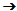.........M(g)...........( metal lattice............free gaseous atoms )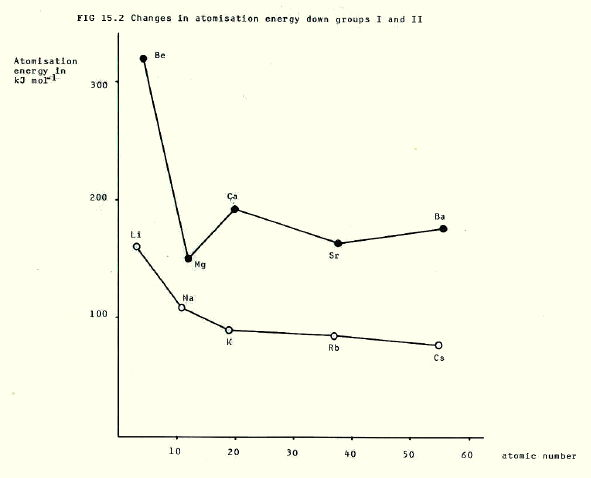In contrast, electrical and thermal conductivity do not follow the pattern that might be predicted from decreasing bond strength. You might predict that it would be easier to transfer electrons through a lattice in which they are are not tightly held. In fact it is more difficult, a bit like trying to push a car made of candyfloss. Thus conductivity decreases down both groups and is generally greater in group II.

15.2.3. Ionisation becomes even more able to occur down both groups as the outer electrons become further from the attractive force of the nucleus. The higher effective nuclear charge makes ionisation generally less likely in group II, though it is hardly relevant in practice.

Both these facts are reflected in the values of the first ionisation energies which decrease down both groups, and which are generally higher in group II: the more stongly an electron is attracted by the nucleus, the greater the energy needed to remove it.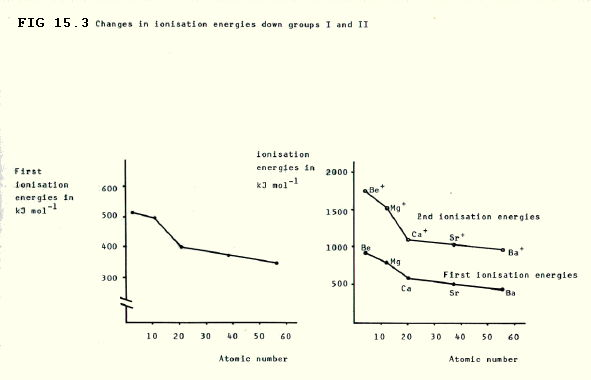Remember that first ionisation energy is the energy change involved in the process:

M(g)....................M+(g)..........+..........e-

15.2.4. Flame tests are also a characteristic of the ease with which electrons are promoted to higher energy levels. The heat of a flame is enough to raise electrons, even in the ions, to higher levels. When they return to lower levels, electromagnetic radiation is emitted (section 2.2.).

In the case of group I and II elements, some of these emissions are in the visible light range:

TABLE 15.1. Flame test colours.

 Element Flame Colour Electron Transition (interest only) Lithium red 2p2s Sodium yellow (very strong) 3p3s Potassium lilac 4d4p Calcium brick red 5s4p

15.2.5. The above changes (sections 15.2.1. to 15.2.3.) do not occur smoothly down the groups. The differences are due to the different screening effects of different types of orbital (section 13.2.).

15.2.6. The strength of hydration of the ions decreases down both groups. This is predictable because the increase in size of the ions down the groups means that their surface charge density decreases, and the d- oxygen atoms of water are therfore less strongly attracted.

In group II, the generally smaller size, together with the double positive charge, enables us to predict that the water molecules will be generally attracted more strongly. In some cases hydrolysis occurs (section 14.4.2.ii.).

These facts are reflected in the hydration energies which decrease down the groups, and are generally higher in group II. Remember, hydration energy is the energy change during the following process:

Eg: Group I: ..........M+(g)....................M+(aq)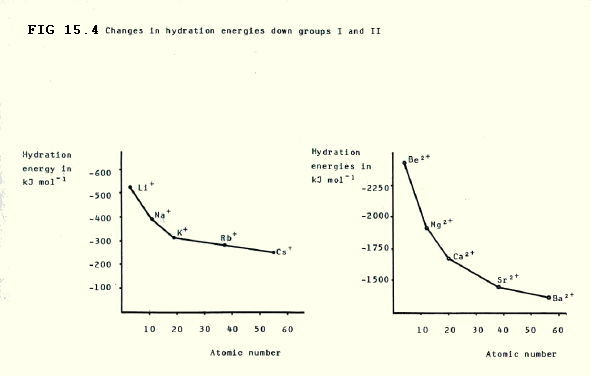15.2.7. Standard electrode potentials numerically increase (become more negative) down both groups. The values in group II are generally lower (less negative). Remember, electrode potentials are quoted for reduction reactions i.e.

M+(aq)..........+..........e-....................M(s)

M2+(aq)..........+..........2e-....................M(s)

TABLE 15.2. Standard electrode potentials for elements in groups I and II.

 Group I elements Group II elements Element S.E.P. Element S.E.P. Li -3.05V Be -1.85V Na -2.71V Mg -2.38V K -2.93V Ca -2.87V Rb -2.95V Sr -2.89V Cs -2.92V Ba -2.90V

The increasing negative values mean the the reverse process (oxidation) becomes even more likely to occur as the groups are descended. Thus the metals become even better reducing agents as the groups are descended. The generally less negative values in group II mean that the oxidation is less likely in group II and the elements are slightly poorer reducing agents.

Note that the redox potential for H2O(l) + e-OH-(aq) + ˝H2(g) is 0.41V, so it can be predicted that all these elements reduce water to hydrogen.

Continuing the emphasis on the reverse process, it can be seen that the change from metal to aqueous metal ions can be broken down into three simpler processes: atomisation, ionisation, and hydration (though it should not be imagined that these three processes occur one after the other).

Down the groups, it is the increasing ability of the elements to be atomised (section 15.2.2.) and their increasing ability to undergo ionisation (15.2.3.) which account for the increasing liklihood of the change from metal to aqueous metal ions. These two increases counteract the decreasing strength with which the ions are hydrated (section 15.2.6.). This also gives a more detailed account of the numerical increase in S.E.P. values.

Similarly, the lower ability of group II metals to undergo atomisation and ionisation (sections 15.2.2. and 15.2.3.) outweigh the greater strength of their hydration (section 15.2.6.). This accounts for the lower liklihood of their change from metal to aqueous metal ions, and their numerically lower redox potentials.

The idiosyncratic S.E.P value for lithium is a charateristic of the particularly small size of its ions and the consequent strength with which water molecules are attracted to them. This outweighs the greater attraction of lithium's nuclei for their outer electrons i.e. the lower ability of the metal to undergo atomisation/ionisation.

Beryllium ions are even smaller and, moreover, doubly charged. However, this does not outweigh the strength of beryllium's metallic bonding and its relative inability to ionise, a process which, it should be remembered, involves removal of two electrons in group II.

15.2.8. Reactivity

i) Reactivity with water generally follows the pattern that might be predicted from the order of the redox potentials shown above i.e. reactivity increases down both groups and is generally lower in group II. Indeed the series in which elements are placed in order of redox potential (electrochemical series) is often known as the reactivity series.

However, some of the rather clumsy language in section 15.2.7. was used in order to avoid using the concept of reactivity. Redox potentials give a measure of how likely a reaction is, that is information about equilibrium, or thermodynamic information (sections 11.13. and 12.5.3.).

In contrast, reactivity is a term most often used in connection with observable rate of reaction, kinetic information. Thermodynamic reactivity is more closely reflected in the amount of heat generated in a reaction, though in table 15.3., even this is confused with the rate at which heat is generated.

In support of this distinction, lithium is much less reactive than the other elements in group I despite the extremely high value of its redox potential.

TABLE 15.3. Reactivity of group I and II elements with water

 Group I elements Group II elements Li: slow reaction with cold water Be: slow reaction with steam Na: molten bead skims on surface, ignition possible Mg: very slow reaction with cold water, steady reaction with steam K: ignition, possible explosion Ca: steady reaction with cold water, fast reaction in hot water Rb: explosive Sr: reacts readily with cold water Cs: explosive Ba: vigorous reaction with cold water

The reason for lithium's low reactivity is the strength of its metallic lattice (section 15.2.2.). Lithium atoms/ions must be pulled away from the lattice for reaction to occur. This, the rate-determining step, is a difficult and slow process. In energy terms, the activation energy is high.

ii) Reactivity with air increases down the groups, and is generally lower in group II. They all tarnish in air, though beryllium and magnesium form only a surface coating.

All the elements burn in air to form oxides. There are three possible oxides, the normal oxide, the peroxide, and the superoxide. Not all the elements form all three oxides on combustion in an adequate supply of air, indeed some of the oxides do not even exist for certain of the elements. The explanation is contained within section 15.3.3.

TABLE 15.4. Oxides of group I and II elements.

 Oxide ion Elements which form it on combustion in adequate supply of air Elements which form it at all Normal O2- Gp I: Li (&Na) GpII: All Gp I: All Gp II: All Peroxide O22- Gp I: Na, (K, Rb & Cs) Gp II: (Sr) & Ba Gp I: All Gp II: All except Be Superoxide O2- Gp I: (Na), K, Rb, Cs Gp II: None Gp I: All except Li Gp II: All except Be & Mg

iii) Reactivity with nitrogen decreases down the groups and is generally greater in group II. This is reflected by the fact that, when they burn in air, group II elements form some nitride, the proportion of which decreases down the group. Moreover, lithium is the only group I element to combine directly with nitrogen.

The reason that group II elements combine directly with nitrogen is that the small size and double charge of their ions enables them to form a very strong lattice with nitride ions. This is strong enough to outweigh the strong triple bond which needs to be broken in nitrogen, as well as the metallic bonding which is broken in the elements themselves.

These predictions are supported by a study of the energy changes involved.

Nitride ions, like hydride ions (section 14.3.2.i.) and oxide ions (sections 14.5.2.i. and 15.3.5.) have such a high surface charge density that they are hydrolysed in water, producing an alkaline solution: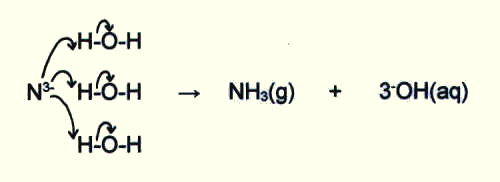15.3. THE COMPOUNDS

15.3.1. Most of the compounds are ionic, though the small size of lithium and beryllium ions gives the compounds of these two elements a high degree of covalent character (section 4.6.2.i.).

15.3.2. The strength of the lattices decreases down both groups, and is generally greater in group II. This is because (as a rough initial guide) the larger the metal ion, the less tightly it can pack with the anions. The forces of attraction are inversely proportional to (r+ + r-)2, where r+ and r- are the radii of the positive and negative ions respectively. Ions of similar size form particularly strong lattices.

Also, the more highly charged the metal ion, the more strongly it attracts the anions i.e. the forces of attraction are proportional to the charge difference between the ions, z.

The changes in lattice strength are reflected by the overall decrease in lattice enthalpies down the groups, and the generally higher lattice enthalpies in group II. Remember that lattice enthalpy is the enthalpy change for the process:

MX(s)....................M+(g)..........+..........X-(g)

though often it is quoted for the reverse process. (In fact, exam boards these days mark as wrong the more consistent (with bond enthalpy) measure of lattice enthalpy as the enthalpy change involved in breaking the lattice.)

Note that lattice enthalpy will be proportional to z/(r+ + r-)

15.3.3. Solubility of compounds shows somewhat complex changes down the group.

The overall process for MX(s) is represented by:

MX(s)....................M+(aq)..........+..........X-(aq)

in other words, breaking of the lattice and hydration of the ions.

Down both groups:

i) The lattice becomes weaker (section 15.3.2.) tending to favour solubility;

ii) Atraction for water molecules (hydration) becomes weaker (section 15.2.6.), tending to discourage solubility.

The key to the balance between these counteracting effects is the size of the anion:

Since the strength of the lattice is inversely proportional to (r+ + r-)2, then:

- for small anions, the decreasing strength of the lattice will be considerable and outweigh the decreasing strength of hydration, thus solubility will increase (e.g. fluorides and hydroxides i.e. F- and OH-).

- for large anions, the decreasing strength of the lattice will be insignificant compared with the decreasing strength of hydration, and solubility will decrease (e.g. sulfates(VI) and carbonates i.e. SO42- and CO32-).

- for intermediate sized anions, the solubility at first decreases, and then increases (e.g. chlorides and nitrates(V) i.e. Cl- and NO3-).

Note that the size of an ammonium ion (NH4+) at 0.143nm is inbetween that of a potassium ion at 0.133nm and that of a rubidium ion at 0.148nm. What would you predict about the solubilities of ammonium compounds? Obviously, potassium salts are more common than rubidium salts and it turns out that ammonium and potassium salts can behave quite similarly.

These group changes are summarised in FIG. 15.5.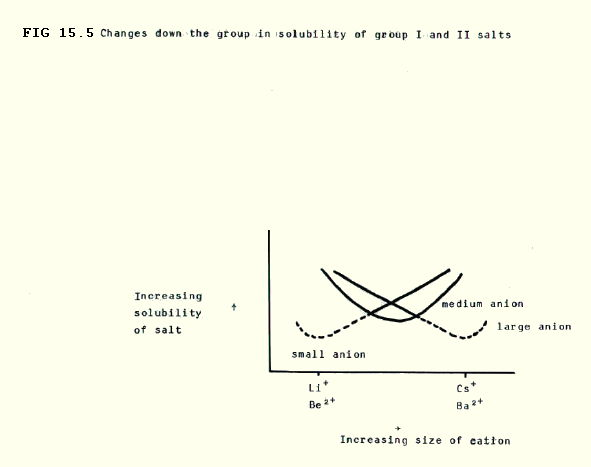For a given anion, the group II compounds are generally less soluble because the effect of the double charge on the strength of the lattice is greater than the effect on hydration.

Moreover, doubly charged cations (group II) form particularly strong lattices with doubly charged anions (e.g. CO32-) so these are generally less soluble than the compounds of group II elements with singly charged anions. This, together with the similarity in size of barium and sulphate ions (section 15.3.2.), accounts for the extreme insolubility of barium sulphate.

Lattice enthalpy and hydration enthalpy data can be used to check predictions. Note also that in border line cases, entropy changes must be considered.

15.3.4. The thermal stability of compounds with large anions increases down both groups, and is generally lower in group II.

Thermal instability of the compounds with large anions is a function of the polarising power of the cation. This in turn is greatest for small ions (section 15.2.1.), and highly charged ions i.e. ions with a high surface charge density. (Hence the group changes and differences described in the last paragraph.)

Thus ions with a high surface charge density tend to polarise large anions, pulling electrons into bonds with themselves, and breaking off a smaller anion which forms a stronger lattice.

### E.g. polarising effect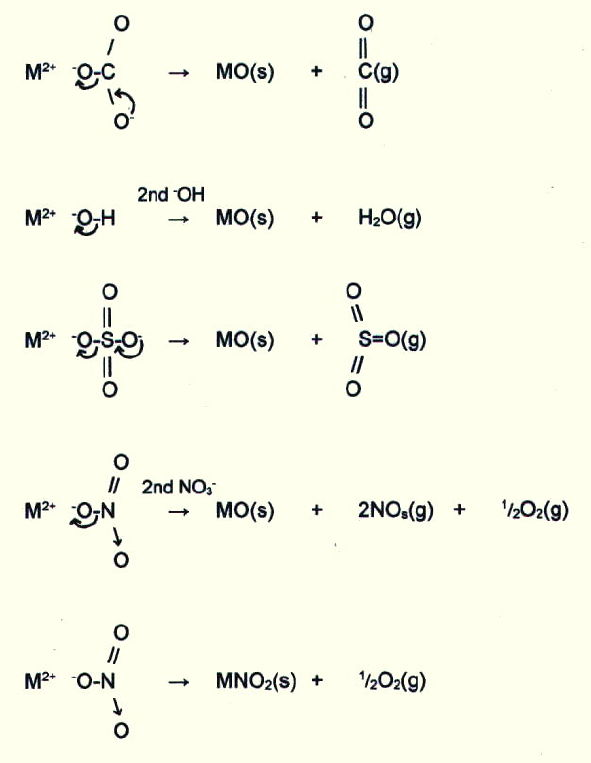Thus the carbonates, hydroxides, and nitrates(III) of group II elements and of lithium in group I tend to decompose in the same way on heating. Thermal stability of the group II carbonates, hydroxides, and nitrates(V), increases down the group. Beryllium sulphate(VI) is the only sulphate(VI) to decompose at comparable temperatures.

The carbonates and hydroxides of group I elements other than lithium are relatively stable to heat, and their nitrates(V) decompose according to the fifth equation in the above list.

The polarising power of cations with a high surface charge density also accounts for the increasing stability down the groups of oxides with large anions, as well as the difficulty in forming group II superoxides at all (section 15.2.8.ii. and table 15.4.).

15.3.5. Acid/base properties of the oxides and hydroxides: With the exception of beryllium, all the group I and group II elements form basic oxides and hydroxides (sections 14.5.2.i. and 14.5.3.i.). Beryllium forms amphoteric oxides and hydroxides (14.5.2.iv. and 14.5.3.iii.).

In general, the oxides react vigorously with water, though BeO is insoluble (section 14.5.2.iv.) and magnesium hardly reacts. There is, then, increasing reactivity down the groups and lower reactivity in group II, as may be predicted. The reaction is between the oxide ions and water, and the vigour depends on the ease with which they are broken away from the lattice (section 15.3.2.)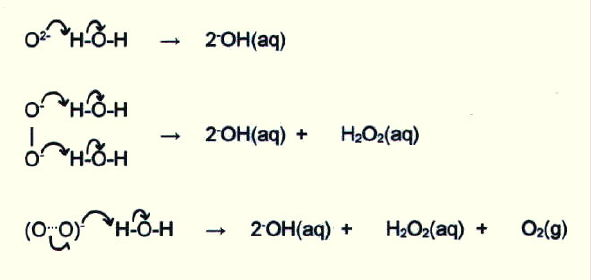Compare this with the reactions of the hydrides (section 14.3.2.i.) and nitrides (section 15.2.8.iii.) in water.

ii) All the group II hydroxides except LiOH are very strong bases and very soluble in water. In water they clearly produce hydroxide ions i.e. an alkaline solution. Solubility increases down the group (section 15.3.3.) and thus, so too, does maximum possible concentration of hydroxide ions (and max. alkalinity).

The group II hydroxides are less soluble (15.3.3.), though solubility again increases down the group and thus, so too, does the maximum possible concentration of hydroxide ions (and max. alkalinity). Beryllium hydroxide is weakly basic and insoluble in water.

The thermal stability of hydroxides has already been discussed in section 15.3.4.

15.3.6. The important properties of other compounds like hydrides and chlorides can be predicted from the relevant parts of sections 14.3. and 14.4. together with an understanding of the changes down and between groups I and II discussed in this chapter.

15.4. QUESTIONS

1) Draw tables summarising the properties of the group I and II elements and their compounds and the changes down both groups plus the differences between the groups. You may need a big piece of paper. E.g.

 PROPERTY GROUP I group differences GROUP II Elements Li.................Cs Be.................Ba E.g. melting point decreases down group greater in group II decreases down group Compounds ..... ..... ..... ..... ..... ..... .....

2) Which will involve the greater contraction in size, the formation of ions by group I elements, or the formation of ions by group II elements?

3) Explain the relationship between changes in metallic bonding down groups I and II, and the enthalpy changes described in section 15.2.2.

4) Describe the relationship between the increasing number of shells down groups I and II, and the numerical increase in standard electrode potentials down the groups. Also account for the anomalous S.E.P. of lithium in terms of its size, and for the difference between groups I and II in terms of the higher effective nuclear charge of group II elements.

5) Predict the differences in standard electrode potentials described in question 4, using predictions about atomisation, ionisation, and hydration enthalpies.

6) Head elements (those at the top of groups) are often said to show anomalous behaviour. List and explain any examples of anomalous behaviour shown by lithium and beryllium and their compounds.

7) Lithium and magnesium show similarities which are often said to be an example of a diagonal relationship. List any examples of such a diagonal relationship, and describe the basis of the effect.

8) If the redox potentials of group I and II elements are not true indicators of their reactivity with water, how should this really be predicted? Apply your method.

9) Write balanced equations for the reactions which occur when each of the following elements is heated in an adequate supply of air: lithium, potassium, beryllium and calcium.

10) Are sections 15.2.8. ii and iii talking about kinetic, or thermodynamic reactivity? Explain.

11) How can lattice enthalpy and hydration enthalpy data be used to check solubility predictions (section 15.3.3.)?

Unless otherwise stated, all materials in this web version of chapter 15 are © 2007 Adrian Faiers MA (Oxon) MCIPR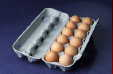What 's the connection between a dozen eggs and a garden mole?Answer: Not a lot, really, but see Chapter 1